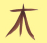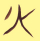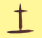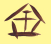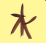# Publications

On this site, I publish documents online.

On the number of monochromatic crossings in rectilinear embeddings of complete graphs
Master's Thesis

This thesis considers the following combinatorial optimization problem in discrete geometry: Given a set of finitely many points in the plane in general position, we want to draw line segments with one of two colors between each two of those points, such that the number of monochromatic crossings, i.e., the number of line segment intersections that are of the same color, is minimized. In this thesis, several approaches will be discussed including an upper bound heuristics by local optimization and a lower bound by linear programming. By this approach, the problem has been solved for up to ten points in the plane by computer.

NAWI Graz, 2019, betreut von Oswin AICHHOLZER
Symmetrische Funktionen
Bachelor's Thesis

Symmetric polyonmials form an interesting subring of a ring of polynomials in several variables. For each bound n ∈ N, the set of symmetric polynomials with degree at most n forms a finite dimensional vector space over the base field. Within this vector space, there are five canonical constructions of a base. In this thesis, these basis constructions are explained and we discribe how to obtain the transformation matrices and discuss some of their properties. So this thesis extends the specalised paper below, where only one line of one matrix was evaluated, but by different methods. In this thesis, we see a more general result that goes back to I. G. Macdonald.

NAWI Graz, 2017, betreut von Karin BAUR
Symmetrische Polynome und deren Eigenschaften unter besonderer Betrachtung der Koeffizienten
Specialised paper

In chapter 1 this paper mentions many properties and theorems of polynomials, furthermore it defines polynomials in more than one variable and the number of partitions of a non-negative integer. This chapter is important to understand the main part, chapter 2. The most important content of this paper are the Newton’s identities (2.2). It gives us an implicit rule to evaluate the coefficients of the polynomial in elementary sym- metric polynomials which equals a power sum. Then we create an explicit formula to evaluate the coefficients out of our implicit rule (theorem 2.2.10). Furthermore we take a look at some special cases where the formula gets even easier (2.3). Section 2.4 deals with ideas for interesting, but yet unsolved problems. Chapter 3 deals with some problems where the results of this paper are useful. In the appendix (6.1) you can see tables where the coefficients of the power sums are calculated up to s10.

BRG Schloss Wagrain, 2011, betreut Rupert SODL